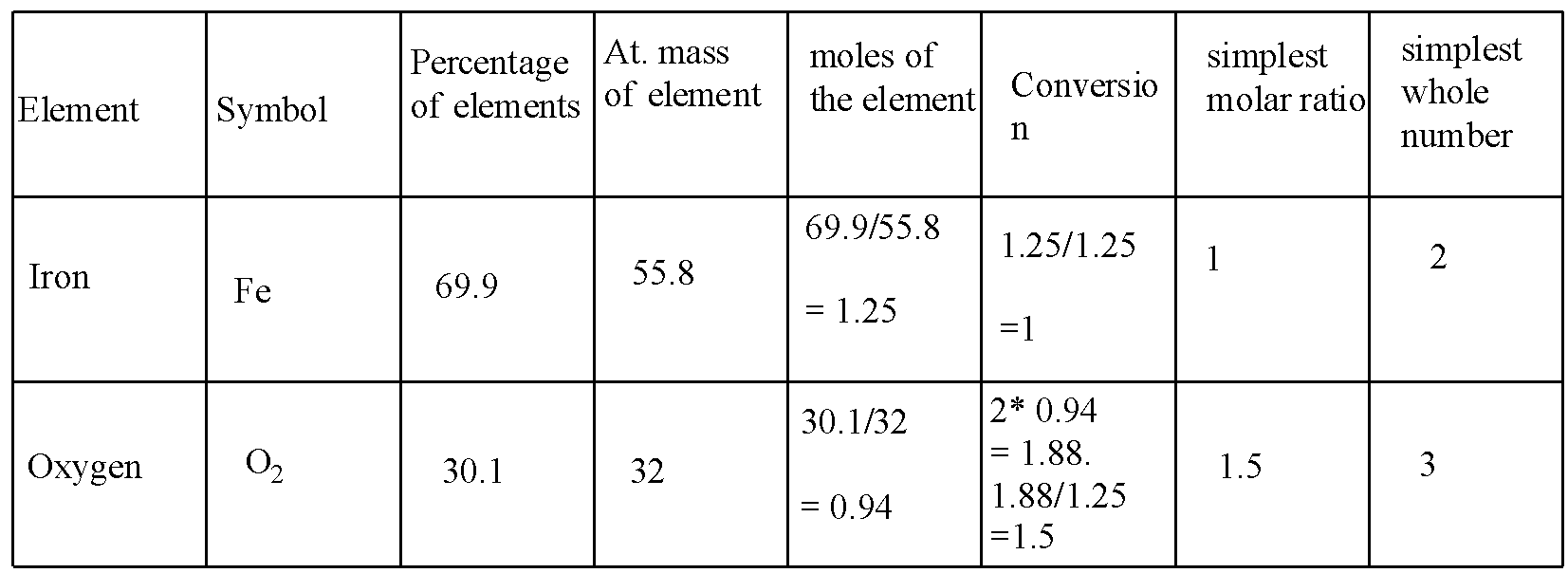QuestionAnswers

# Determine the empirical formula of an oxide of iron which has 69.9% iron and 30.1% dioxygen by mass.

Hint: The empirical formula of a chemical compound is calculated with the help of two factors i.e., the percentage composition of elements and atomic mass of the element.

The empirical formula is the chemical formula that tells the simplest whole-number ratio of the atoms of all elements present in one molecule.
It is deduced by- (a) percentage composition of elements (b)- atomic masses.
There are some steps for calculating the empirical formula:
i)- To convert the mass percent into grams. The given mass percentage represents the masses of the element in grams.
ii)- To calculate the number of moles. Divide the percentage of each element by its atomic mass.
$\text{moles of the element= }\frac{\text{mass of the element}}{\text{At}\text{. mass of the element}}$
iii)-To calculate the simplest molar ration. Divide the moles obtained in step (i) by the smallest quotient or the least value from amongst the values obtained for each element. This gives the simplest molar ratio.
iv)- To calculate the simplest whole number ratio. The simplest molar ratios, as calculated in step 2, are generally whole numbers. If not, then (a) raise the values to the nearest whole number, or (b) multiply the simplest atomic ratio by a suitable integer.
v)- To write the empirical formula. Insert the numerical value of the simplest whole number.
According to the following steps, for iron and oxygen is solved in the table:In the conversion, the 0.94 is multiplied with 2 because the oxygen is diatomic.
Hence, the formula is $F{{e}_{2}}{{O}_{3}}$ .

Note:
The simplest molecular ration should always be converted into the whole number. If not converted this would give the exact or true formula which gives the molecular formula. Don't get confused between molecular and empirical formulas.